# High School Math : Multiplying and Dividing Exponents

## Example Questions

### Example Question #1 : Simplifying Exponents

Simplify the following expression.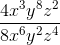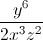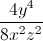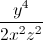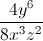Explanation:

When dividing with exponents, the exponent in the denominator is subtracted from the exponent in the numerator. For example: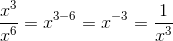.

In our problem, each term can be treated in this manner. Remember that a negative exponent can be moved to the denominator.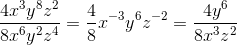Now, simplifly the numerals.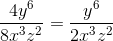### Example Question #2 : Simplifying Exponents

Simplify the following expression.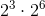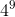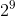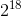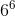Explanation:

We are given:Recall that when we are multiplying exponents with the same base, we keep the base the same and add the exponents.

Thus, we have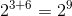.

### Example Question #1 : Simplifying Exponents

Simplify the following expression.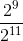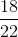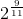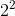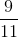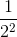Explanation:

Recall that when we are dividing exponents with the same base, we keep the base the same and subtract the exponents.

Thus, we have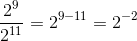.

We also recall that for negative exponents,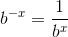.

Thus,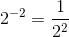.

### Example Question #4 : Simplifying Exponents

Simplify the following exponent expression: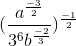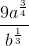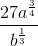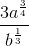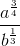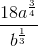Explanation:

Begin by rearranging the terms in the numerator and denominator so that the exponents are positive: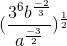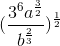Multiply the exponents: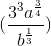Simplify:### Example Question #5 : Simplifying Exponents

Simplify the expression: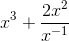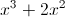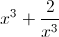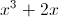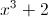Explanation:

First simplify the second term, and then combine the two: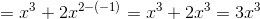### Example Question #6 : Simplifying Exponents

Solve for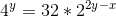Cannot be determined from the given information.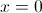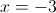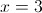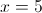Explanation:

Rewrite each side of the equation to only use a base 2: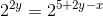The only way this equation can be true is if the exponents are equal.

So: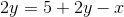The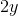on each side cancel, and moving theto the left side, we get: